# Song and Mason equation of state for hard convex bodies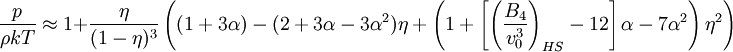$\frac{p}{\rho kT} \approx 1 + \frac{\eta}{(1-\eta)^3}\left((1+3\alpha)-(2+3\alpha-3\alpha^2)\eta + \left(1+\left[\left(\frac{B_4}{v_0^3}\right)_{HS} -12\right] \alpha -7\alpha^2\right)\eta^2\right)$
where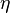$\eta$ is the packing fraction, given by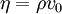$\eta=\rho v_0$ where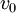$v_0$ is the volume of one particle, and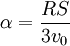$\alpha= \frac{RS}{3v_0}$
where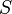$S$, the surface area, and$R$ the mean radius of curvature.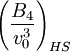$\left(\frac{B_4}{v_0^3}\right)_{HS}$ is the fourth virial coefficient for the hard sphere model.